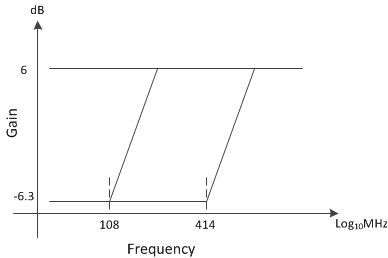JAJSDO4C February   2017  – April 2020

PRODUCTION DATA.

1. 特長
2. アプリケーション
3. 概要
4. 改訂履歴
5. 概要（続き）
6. Device Comparison Table
7. Pin Configuration and Functions
8. Specifications
9. Detailed Description
1. 9.1 Overview
2. 9.2 Functional Block Diagrams
3. 9.3 Feature Description
4. 9.4 Device Functional Modes
5. 9.5 Register Maps
10. 10Application and Implementation
1. 10.1 Application Information
2. 10.2 Typical Application
11. 11Power Supply Recommendations
12. 12Layout
13. 13デバイスおよびドキュメントのサポート
14. 14メカニカル、パッケージ、および注文情報

• AAV|144

#### 9.3.4 SerDes Equalizer

All channels of the DAC38RF82 (or DAC38RF89) incorporate an adaptive equalizer, which can compensate for channel insertion loss by attenuating the low frequency components with respect to the high frequency components of the signal, thereby reducing inter-symbol interference. Figure 29 shows the response of the equalizer, which can be expressed in terms of the amount of low frequency gain and the frequency up to which this gain is applied (i.e., the frequency of the ’zero’). Above the zero frequency, the gain increases at 6 dB/octave until it reaches the high frequency gain.Figure 29. Equalizer Frequency Response

The equalizer can be configured via fields EQ and EQHLD in register SRDS_CFG1 (8.5.86). Table 6 and Table 7 summarize the options. When enabled, the receiver equalization logic analyzes data patterns and transition times to determine whether the low frequency gain should be increased or decreased. The decision logic is implemented as a voting algorithm with a relatively long analysis interval. The slow time constant that results reduces the probability of incorrect decisions but allows the equalizer to compensate for the relatively stable response of the channel. The lock time for the adaptive equalizer is data dependent, and so it is not possible to specify a generally applicable absolute limit. However, assuming random data, the maximum lock time will be 6x106 divided by the CDR activity level. For field CDR in register SRDS_CFG1 (8.5.86) = 110, the activity level is 1.5 x 106 UI.

When EQ = 0, finer control of gain boost is available using the EQBOOST IEEE1500 tuning chain field, as shown in Table 6.

### Table 6. Receiver Equalization Configuration

EQ EFFECT
[1-0] 00 No equalization. The equalizer provides a flat response at the maximum gain. This setting may be appropriate if jitter at the receiver occurs predominantly as a result of crosstalk rather than frequency dependent loss.
01 Fully adaptive equalization. The zero position is determined by the selected operating rate, and the low frequency gain of the equalizer is determined algorithmically by analyzing the data patterns and transition positions in the received data. This setting should be used for most applications.
10 Precursor equalization analysis. The data patterns and transition positions in the received data are analyzed to determine whether the transmit link partner is applying more or less precursor equalization than necessary.
11 Postcursor equalization analysis. The data patterns and transition positions in the received data are analyzed to determine whether the transmit link partner is applying more or less post-cursor equalization than necessary.
 0 Default
1 Boost. Equalizer gain boosted by 6 dB, with a 20% reduction in bandwidth, and an increase of 5mW power consumption. May improve performance over long links.

### Table 7. Receiver Equalizer Hold

EQHOLD EFFECT
0 Equalizer adaption enabled. The equalizer adaption and analysis algorithm is enabled. This should be the default state.
1 Equalizer adaption held. The equalizer is held in its current state. Additionally, the adaption and analysis algorithm is reset.

### Table 8. Relationship Between Lane Rate and SerDes PLL Output Frequency

EQBOOST GAIN BOOST (dB) BANDWIDTH CHANGE (%) POWER INCREASE (mW)
00 0 0 0
01 2 -30 0
01 4 10 5
11 6 -20 5

When EQ is set to 010 or 011, the equalizer is reconfigured to provide analytical data about the amount of pre and post cursor equalization respectively present in the received signal. This can in turn be used to adjust the equalization settings of the transmitting link partner, where a suitable mechanism for communicating this data back to the transmitter exists. Status information is provided by setting field DTEST in register DTEST (8.5.76) to “0111” for EQOVER and “0110” for EQUNDER. The procedure is as follows:

1. Enable the equalizer by setting fields EQHLD low and EQ to “001” (register SRDS_CFG1 8.5.86). Allow sufficient time for the equalizer to adapt;
2. Set EQHLD to 1 to lock the equalizer and reset the adaption algorithm. This also causes both EQOVER and EQUNDER to become low;
3. Wait at least 48 UI, and proportionately longer if the CDR activity is less than 100%, to ensure the 1 on EQHLD is sampled and acted upon;
4. Set EQ to “010” or “011”, and EQHLD to 0. The equalization characteristics of the received signal are analysed (the equalizer response will continue to be locked);
5. Wait at least 150×103 UI to allow time for the analysis to occur, proportionately longer if the CDR activity is less than 100%;
6. Examine EQOVER and EQUNDER for results of analysis
• If EQOVER is high, it indicates the signal is over equalized;
• If EQUNDER is high, it indicates the signal is under equalized;
7. Set EQHLD to 1;
8. Repeat items 3–7 if required;
9. Set EQ to “001”, and EQHLD to 0 to exit analysis mode and return to normal adaptive equalization.

NOTE

When changing EQ from one non-zero value to another, EQHLD must already be 1. If this is not the case, there is a chance the equalizer could be reset by a transitory input state (i.e., if EQ is momentarily 000). EQHLD can be set to 0 at the same time as EQ is changed.

As the equalizer adaption algorithm is designed to equalize the post cursor, EQOVER or EQUNDER will only be set during post cursor analysis if the amount of post cursor equalization required is more or less than the adaptive equalizer can provide.